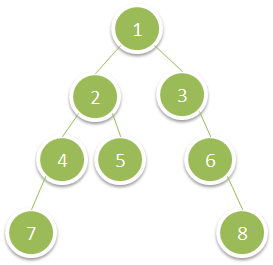# 1302. Deepest Leaves Sum

Given the `root` of a binary tree, return  the sum of values of its deepest leaves.

Example 1:``````Input: root = [1,2,3,4,5,null,6,7,null,null,null,null,8]
Output: 15
``````

Example 2:

``````Input: root = [6,7,8,2,7,1,3,9,null,1,4,null,null,null,5]
Output: 19
``````

Constraints:

• The number of nodes in the tree is in the range `[1, 104]`.
• `1 <= Node.val <= 100`

``````class Solution {
public:
int deepestLeavesSum(TreeNode* root) {
int res = 0;
queue<TreeNode*> q{{root}};
while (!q.empty()) {
int sum = 0;
for (int i = q.size(); i > 0; --i) {
TreeNode *t = q.front(); q.pop();
if (!t->left && !t->right) sum += t->val;
if (t->left) q.push(t->left);
if (t->right) q.push(t->right);
}
res = sum;
}
return res;
}
};
``````

``````class Solution {
public:
int deepestLeavesSum(TreeNode* root) {
int res = 0, mxLevel = 0;
dfs(root, 0, mxLevel, res);
return res;
}
void dfs(TreeNode* node, int level, int& mxLevel, int& res) {
if (!node) return;
if (level > mxLevel) {
mxLevel = level;
res = 0;
}
if (level == mxLevel) {
res += node->val;
}
dfs(node->left, level + 1, mxLevel, res);
dfs(node->right, level + 1, mxLevel, res);
}
};
``````

``````class Solution {
public:
int deepestLeavesSum(TreeNode* root) {
vector<int> sums;
dfs(root, 0, sums);
return sums.back();
}
void dfs(TreeNode* node, int level, vector<int>& sums) {
if (!node) return;
if (level == sums.size()) {
sums.push_back(node->val);
} else {
sums[level] += node->val;
}
dfs(node->left, level + 1, sums);
dfs(node->right, level + 1, sums);
}
};
``````

Github 同步地址:

https://github.com/grandyang/leetcode/issues/1302

https://leetcode.com/problems/deepest-leaves-sum/

https://leetcode.com/problems/deepest-leaves-sum/discuss/463239/JavaC%2B%2BPython-Level-Traversal

https://leetcode.com/problems/deepest-leaves-sum/discuss/565187/Java-Recursive-faster-than-100.00

https://leetcode.com/problems/deepest-leaves-sum/discuss/1152922/JS-Python-Java-C%2B%2B-or-Easy-BFS-or-Recursive-DFS-Solutions-w-Explanation

LeetCode All in One 题目讲解汇总(持续更新中…)

（欢迎加入博主的知识星球，博主将及时答疑解惑，并分享刷题经验与总结，快快加入吧～）|

Venmo 打赏—|—

 微信打赏Venmo 打赏（欢迎加入博主的知识星球，博主将及时答疑解惑，并分享刷题经验与总结，试运营期间前五十位可享受半价优惠～）×

Help us with donation